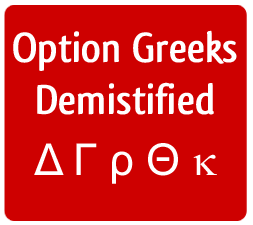# Overview of Option Greeks

Option Greeks are the various partial derivatives of the Black-Scholes Option Pricing Model, each with respect to a different variable. Greeks provide a way to measure the sensitivity of the option price to various factors, such as the underlying asset price, time, volatility, etc. The five key greeks commonly used by the traders are:\Delta

Delta measures the sensitivity of an option's value to a change in the price of the underlying asset. It indicates how much the value of an option will change with a one dollar change in the price of the underlying stock.

Gamma

Gamma is a measure of delta's sensitivity to changes in the price of the underlying asset. Gamma is the first derivative of delta with respect to the stock price. Gamma is also called curvature.

Theta

Theta is a measure of the sensitivity of an option to the time remaining until expiration. It is a measure of the time decay of the option. The value of theta is the dollar value that the option will lose each day with the passage of time.

Vega

Vega measures the sensitivity of the price of an option to changes in volatility. An increase in volatility increases the price of all options, and a decrease in volatility decreases the price of all options. Vega measures how much the value of the option will increase or decrease with change in volatility.

Rho